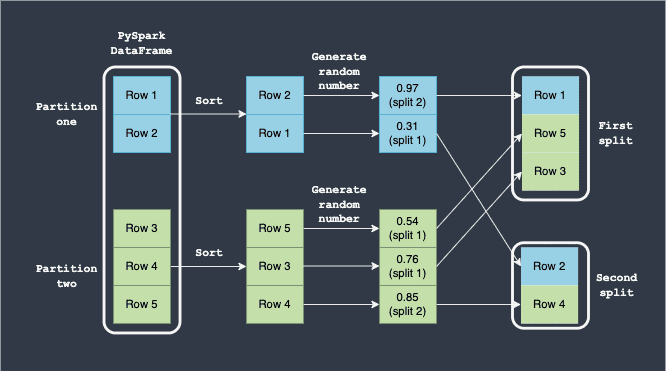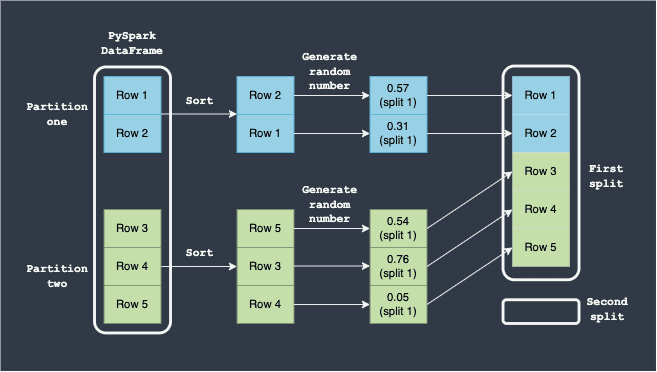search
Search
Unlock 100+ guides
search toc
close
Cancel
Post
account_circle
Profile
exit_to_app
Sign out
search
keyboard_voice
close
Searching Tips
Search for a recipe:
"Creating a table in MySQL"
Search for an API documentation: "@append"
Search for code: "!dataframe"
Apply a tag filter: "#python"
Useful Shortcuts
/ to open search panel
Esc to close search panel
to navigate between search results
d to clear all current filters
Enter to expand content previewDoc SearchCode Search BetaSORRY NOTHING FOUND!
mic
Start speaking...Voice search is only supported in Safari and Chrome.
Shrink
Navigate to

# PySpark DataFrame | randomSplit method

schedule Aug 12, 2023
Last updated
local_offer
PySpark
Tags
mode_heat
Master the mathematics behind data science with 100+ top-tier guides
Start your free 7-days trial now!

PySpark DataFrame's `randomSplit(~)` method randomly splits the PySpark DataFrame into a list of smaller DataFrames using Bernoulli sampling.

# Parameters of randomSplit

1. `weights` | `list` of `numbers`

The list of weights that specify the distribution of the split. For instance, setting `[0.8,0.2]` will split the PySpark DataFrame into 2 smaller DataFrames using the following logic:

• a random number is generated between 0 and 1 for each row of the original DataFrame.

• we set 2 acceptance ranges:

• if the random number is between 0 and 0.8, then the row will be placed in the first sub-DataFrame

• if the random number is between 0.8 and 1.0, then the row will be placed in the second sub-DataFrame

The following diagram shows how the split is performed:Here, note the following:

• we assume that the PySpark DataFrame has two partitions (blue and green).

• the rows are first locally sorted based on some column value in each partition. This sorting guarantees that as long as the same rows are in each partition (regardless of their ordering), we would always end up with the same deterministic ordering.

• for each row, a random number between 0 and 1 is generated.

• the acceptance range of the first split is `0` to `0.8`. Any row whose generated random number is between `0` and `0.8` will be placed in the first split.

• the acceptance range of the second split is `0.8` to `1.0`. Any row whose generated random number is between `0.8` and `1.0` will be placed in the second split.

What's important here is that there is never a guarantee that the first DataFrame will have 80% of the rows, and the second will have 20%. For instance, suppose the random number generated for each row falls between `0` and `0.8` - this means that none of the rows will end up in the second DataFrame split:On average, we should expect that the first DataFrame will have 80% of the rows while the second DataFrame with 20% of the rows, but the actual split may be very different.

NOTE

If the values do not add up to one, then they will be normalized.

2. `seed` | `int` | `optional`

Calling the function with the same seed will always generate the same results. There is a caveat to this as we shall see later.

# Return value of randomSplit

A list of PySpark DataFrames.

# Examples

Consider the following PySpark DataFrame:

``` df = spark.createDataFrame([["Alex", 20], ["Bob", 30], ["Cathy", 40], ["Dave", 40]], ["name", "age"])df.show() +-----+---+| name|age|+-----+---+| Alex| 20|| Bob| 30||Cathy| 40|| Dave| 40|+-----+---+ ```

## Randomly splitting a PySpark DataFrame into smaller DataFrames

To randomly split this PySpark DataFrame into 2 sub-DataFrames with a 75-25 row split:

``` list_dfs = df.randomSplit([0.75,0.25], seed=24)for _df in list_dfs: _df.show() +-----+---+| name|age|+-----+---+| Alex| 20||Cathy| 40|+-----+---++----+---+|name|age|+----+---+| Bob| 30||Dave| 40|+----+---+ ```

Even though we expect the first DataFrame to contain 3 rows while the second DataFrame to contain 1 row, we see that split was a 50-50. This is because, as discussed above, `randomSplit(~)` is based on Bernoulli sampling.

## Quirks of the seed parameter

The `seed` parameter is used for reproducibility. For instance, consider the following PySpark DataFrame:

``` df = spark.createDataFrame([["Alex", 20], ["Bob", 30], ["Cathy", 40], ["Dave", 40]], ["name", "age"])df +-----+---+| name|age|+-----+---+| Alex| 20|| Bob| 30||Cathy| 40|| Dave| 40|+-----+---+ ```

Running the `randomSplit(~)` method with the same seed will guarantee the same splits given that the PySpark DataFrame is partitioned in the exact same way:

``` list_dfs = df.randomSplit([0.75,0.25], seed=24)for _df in list_dfs: _df.show() +-----+---+| name|age|+-----+---+| Alex| 20||Cathy| 40|+-----+---++----+---+|name|age|+----+---+| Bob| 30||Dave| 40|+----+---+ ```

Running the above multiple times will always yield the same splits since the partitioning of the PySpark DataFrame is the same.

We can see how the rows of a PySpark DataFrame are partitioned by converting the DataFrame into a RDD, and then using the `glom()` method:

``` df = spark.createDataFrame([["Alex", 20], ["Bob", 30], ["Cathy", 40], ["Dave", 40]], ["name", "age"])df.rdd.glom().collect() [[], [Row(name='Alex', age=20)], [], [Row(name='Bob', age=30)], [], [Row(name='Cathy', age=40)], [], [Row(name='Dave', age=40)]] ```

Here, we see that our PySpark DataFrame is split into 8 partitions but half of them are empty.

Let's change the partitioning using `repartition(~)`:

``` df = df.repartition(2)df.rdd.glom().collect() [[Row(name='Alex', age=20), Row(name='Bob', age=30), Row(name='Cathy', age=40), Row(name='Dave', age=40)], []] ```

Even though the content of the DataFrame is the same, we now only have 2 partitions instead of 8 partitions.

Let's call `randomSplit(~)` with the same seed (`24`) as before:

``` list_dfs = df.randomSplit([0.75,0.25], seed=24)for _df in list_dfs: _df.show() +-----+---+| name|age|+-----+---+| Alex| 20|| Bob| 30||Cathy| 40|| Dave| 40|+-----+---++----+---+|name|age|+----+---++----+---+ ```

Notice how even though we used the same seed, we ended up with a different split. This confirms that the `seed` parameter only guarantees consistent splits only if the underlying partition is the same. You should be cautious of this behaviour because partitions can change after a shuffle operation (e.g. `join(~)` and `groupBy(~)`).

thumb_up
thumb_down
Comment
Citation
Ask a question or leave a feedback...
thumb_up
4
thumb_down
0
chat_bubble_outline
0
settings
Enjoy our search
Hit / to insta-search docs and recipes!